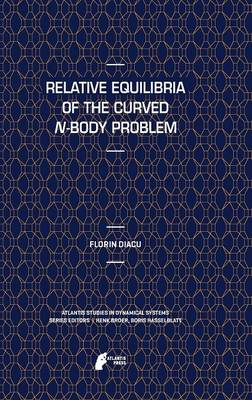•# Relative Equilibria of the Curved N-Body Problem - Atlantis Studies in Dynamical Systems 1 (Hardback)

(author)
£85.50
Hardback 146 Pages / Published: 18/08/2012
• We can order this

Usually dispatched within 3 weeks

The guiding light of this monograph is a question easy to understand but difficult to answer: {What is the shape of the universe? In other words, how do we measure the shortest distance between two points of the physical space? Should we follow a straight line, as on a flat table, fly along a circle, as between Paris and New York, or take some other path, and if so, what would that path look like? If you accept that the model proposed here, which assumes a gravitational law extended to a universe of constant curvature, is a good approximation of the physical reality (and I will later outline a few arguments in this direction), then we can answer the above question for distances comparable to those of our solar system. More precisely, this monograph provides a mathematical proof that, for distances of the order of 10 AU, space is Euclidean. This result is, of course, not surprising for such small cosmic scales. Physicists take the flatness of space for granted in regions of that size. But it is good to finally have a mathematical confirmation in this sense. Our main goals, however, are mathematical. We will shed some light on the dynamics of N point masses that move in spaces of non-zero constant curvature according to an attraction law that naturally extends classical Newtonian gravitation beyond the flat (Euclidean) space. This extension is given by the cotangent potential, proposed by the German mathematician Ernest Schering in 1870. He was the first to obtain this analytic expression of a law suggested decades earlier for a 2-body problem in hyperbolic space by Janos Bolyai and, independently, by Nikolai Lobachevsky. As Newton's idea of gravitation was to introduce a force inversely proportional to the area of a sphere the same radius as the Euclidean distance between the bodies, Bolyai and Lobachevsky thought of a similar definition using the hyperbolic distance in hyperbolic space. The recent generalization we gave to the cotangent potential to any number N of bodies, led to the discovery of some interesting properties. This new research reveals certain connections among at least five branches of mathematics: classical dynamics, non-Euclidean geometry, geometric topology, Lie groups, and the theory of polytopes.

ISBN: 9789491216671
Number of pages: 146
Weight: 412 g
Dimensions: 235 x 155 x 11 mm
Edition: 2012 ed.

MEDIA REVIEWS

From the reviews:

"The book is divided into 5 parts with several chapters and sections in each. ... The book is clear, well written, interesting and easy to read. ... The book is an invitation to more research on this topic and it is a nice source of new problems, particularly for people working in celestial mechanics, dynamical systems, numerical analysis and geometric mechanics. I enjoyed reading it." (Ernesto Perez-Chavela, Mathematical Reviews, June, 2013)